# 预测建模中的正则化与扩增

## 什么是正则化？

• 它消除了预测因子间多重共线性带来的病态。

• 如果最小二乘问题是病态的，例如如果数据点的数量少于特征的数量，那么 lambda 将选择唯一的解。

• 它为提高线性模型的泛化能力提供了一种途径。

## 什么是扩增？

MODEL_QUANTILE 和 MODEL_PERCENTILE 的扩增是数据扩增的一个简单示例：预测因子扩展到更高阶的多项式。在 Tableau 中，预测建模函数内置了几种类型的多项式加法。

• 对于有序维度勒让德多项式高达 3 阶，使得线性模型能够获得增强预测因子和响应之间的二次和三次关系。

• 对于度量，二次埃尔米特多项式允许线性模型获得增强预测器和响应之间的二次关系。

## 在计算中配置 lambda 和扩增

`MODEL_QUANTILE("model=rl, lambda=0.05", 0.5, SUM([Profit]), "augmentation=off", SUM([Sales]))`

 扩增 Lambda 岭回归 是 是 线性回归 是 不适用

## 正则化和扩增注意事项

• 如果您的数据模型错误，则更改正则化参数或扩增不太可能产生明显更好的效果。考虑查看数据类型是否正确（度量与维度）。例如，如果基础数据是时间系列，请考虑使用高斯过程回归，方法是使用 model=gp 更改表计算中的模型。

• 由于 OLS 未正则化，因此没有可以更改的 lambda 值。

• 如果您的数据集非常小，并且您具有维度（尤其是高基数维度），则考虑通过在表中添加 model=rl 来使用岭回归。

• 在所有条件相同的情况下（对于相同的数据集，如果启用或禁用扩增），较低的 lambda 可能会改善拟合，但会损害泛化（导致过度拟合）。

• 相反，高 lambda 可能会将拟合推到一个恒定模型，而不依赖于任何预测因子。这将减少模型容量（导致欠拟合）。

### 示例 1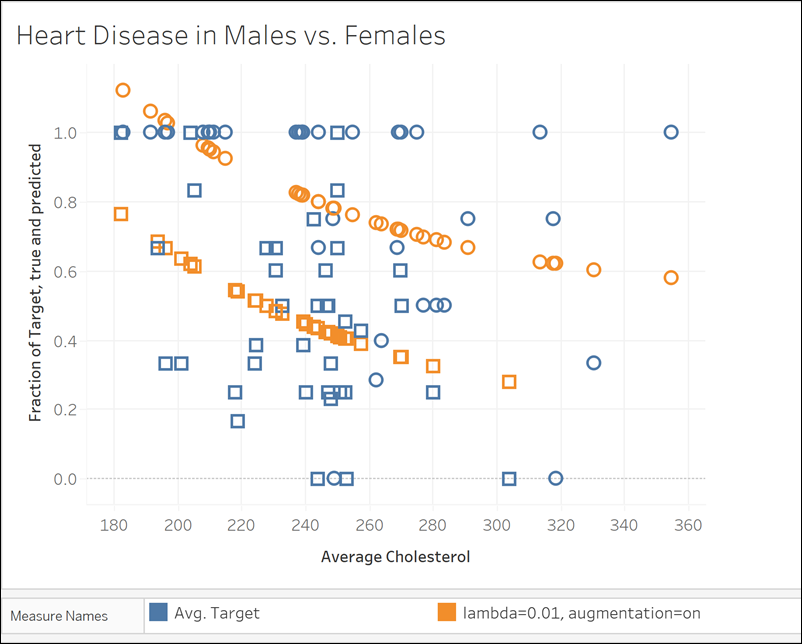MODEL_QUANTILE("model=rl, lambda=0.01", 0.5, AVG([Target]), ATTR([Sex]), "augmentation=on", AVG([Chol]))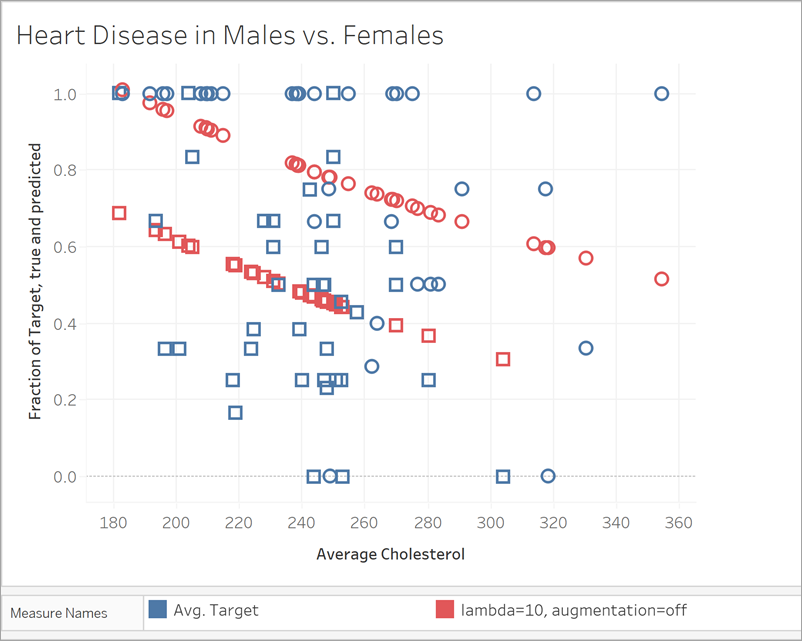MODEL_QUANTILE("model=rl, lambda=10", 0.5, AVG([Target]), ATTR([Sex]), "augmentation=off", AVG([Chol]))

### 示例 2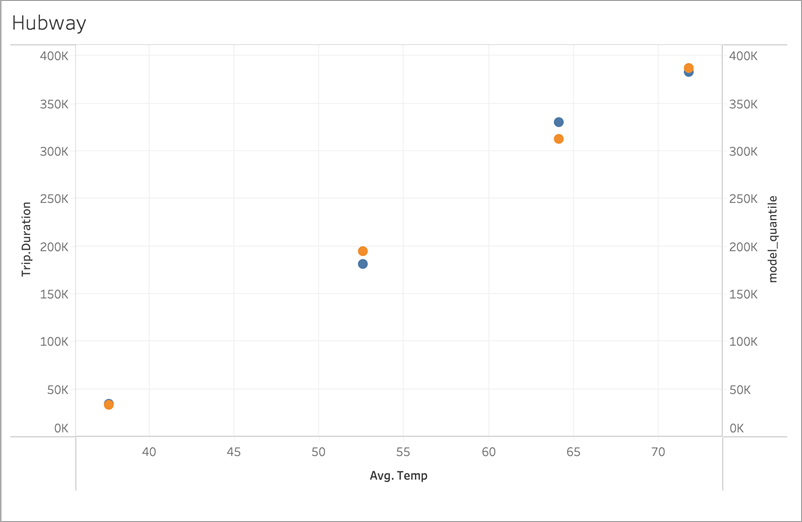MODEL_QUANTILE('model=rl, lambda=0.05', 0.5, sum([Trip.Duration]), AVG([Temp]))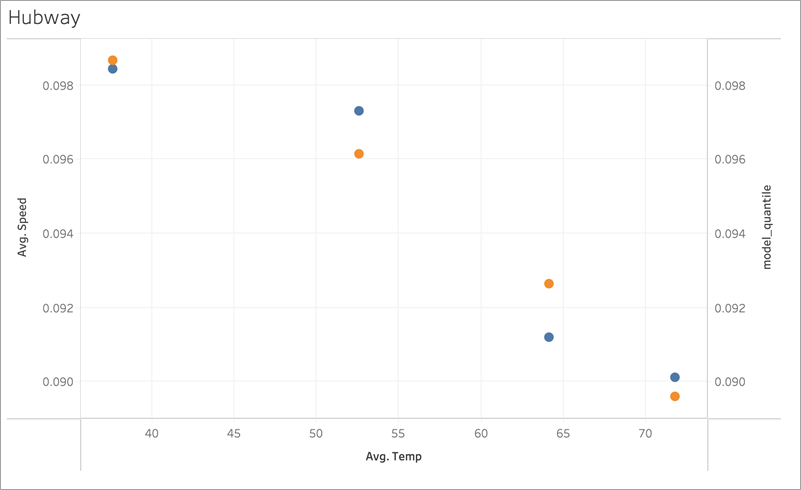MODEL_QUANTILE('model=rl, lambda=0.05', 0.5, AVG([Speed]), AVG([Temp]))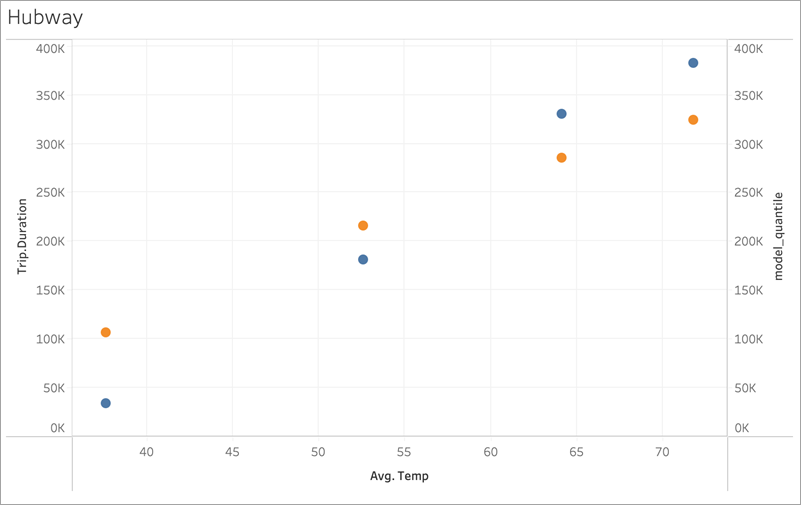MODEL_QUANTILE('model=rl, lambda=2', 0.5, sum([Trip.Duration]), AVG([Temp]))